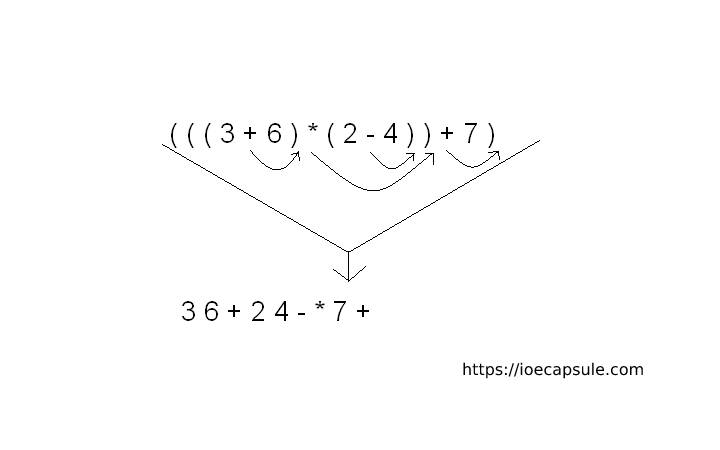### Inflix to Postflix conversion using StackData Structures

Stack is a linear data structure which follows a particular order in which the operations are performed. The order may be LIFO(Last In First Out) or FILO(First In Last Out).

Mainly the following three basic operations are performed in the stack:

• Push: Adds an item in the stack. If the stack is full, then it is said to be an Overflow condition.
• Pop: Removes an item from the stack. The items are popped in the reversed order in which they are pushed. If the stack is empty, then it is said to be an Underflow condition.
• Peek or Top: Returns top element of stack.
• isEmpty: Returns true if stack is empty, else false.

Infix expression:The expression of the form a op b. When an operator is in-between every pair of operands.

Postfix expression:The expression of the form a b op. When an operator is followed for every pair of operands.

Why postfix representation of the expression?
The compiler scans the expression either from left to right or from right to left.

Algorithm
1. Scan the infix expression from left to right.
2. If the scanned character is an operand, output it.
3. Else,
…..3.1 If the precedence of the scanned operator is greater than the precedence of the operator in the stack(or the stack is empty or the stack contains a ‘(‘ ), push it.
…..3.2 Else, Pop all the operators from the stack which are greater than or equal to in precedence than that of the scanned operator. After doing that Push the scanned operator to the stack. (If you encounter parenthesis while popping then stop there and push the scanned operator in the stack.)
4. If the scanned character is an ‘(‘, push it to the stack.
5. If the scanned character is an ‘)’, pop the stack and and output it until a ‘(‘ is encountered, and discard both the parenthesis.
6. Repeat steps 2-6 until infix expression is scanned.
7. Print the output
8. Pop and output from the stack until it is not empty.

Example Using C++

``````
#include<iostream>
#include<cstring>
#include<stack>
using namespace std;

class Inflix{
private:
string expr;
public:
Inflix(){}

Inflix(string expr){
this->expr = expr;
}

Inflix(const Inflix& inflix){
this->expr = inflix.expr;
}

void set(const char* expr){
this->expr = expr;
}

string expression(){
return expr;
}

};

class Postflix{
private:
string expr;

int precedence(char a){
if(a == '+' || a == '-'){
return 1;
}else if(a == '*' || a == '/'){
return 2;
}else if(a == '^'){
return 3;
}else return -1;
}

bool isOperand(char c){
return isalpha(c);
}
public:
Postflix(){}

Postflix(string expr){
this->expr = expr;
}

Postflix(const Postflix& postflix){
this->expr = postflix.expr;
}

Postflix(Inflix& inflix){
//conversion goes here
stack<char> st;
char temp;
for(int i = 0; i < inflix.expression().length(); i++){
temp = inflix.expression()[i];
if(isOperand(temp)){
this->expr+=temp;
}else if(temp == '('){
st.push(temp);
}else if(temp == ')'){
while(!st.empty() && st.top() != '('){
char c = st.top();
st.pop();
this->expr+=c;
}
st.pop();
}else{
//working on operator
while(!st.empty() && precedence(temp) <= precedence(st.top())){
char c = st.top();
st.pop();
this->expr+=c;
}
st.push(temp);
}
}

//clearing remaining stack content
while(!st.empty()){
char ch = st.top();
st.pop();
this->expr+=ch;
}
}

string expression(){
return expr;
}

};

int main(){
string expr;
cout<<"enter expression"<<endl;
cin>>expr;
Inflix inflix(expr);
Postflix postflix = inflix;
cout<<"Postflix: "<<postflix.expression()<<endl;
return 0;
}``````